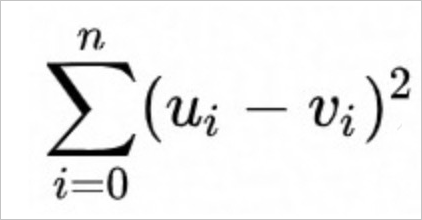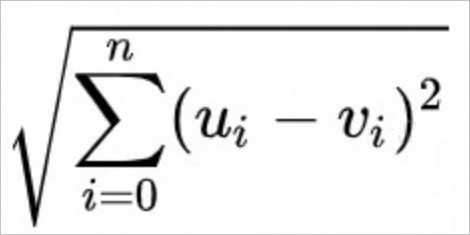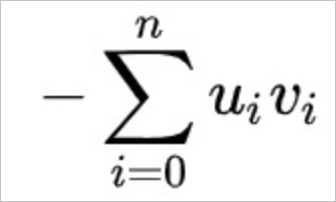Proxima is a high-performance software library that is applied to the big data field. It allows you to search for the nearest neighbors of vectors. Compared with open source software such as Facebook AI Similarity Search (Fassi), Proxima provides higher stability and performance. Proxima provides industry-specific basic modules that allow you to search for similar images, videos, or human faces. Hologres is deeply integrated with Proxima to provide a high-performance vector search service. This topic describes how to perform vector processing in Hologres.

## Use Proxima to perform vector processing

To perform vector processing by using Proxima, perform the following steps:

1. Install the Proxima plug-in.
Proxima is connected to Hologres as an extension. You can run the following command to install the Proxima plug-in:
``create extension proxima;``
The Proxima plug-in works at the database level. You need to install it only once for each database.
2. Search for the nearest neighbors of vectors.
In Hologres, vectors are arrays of FLOAT4 elements. You can search for the nearest neighbors of vectors by calling the following functions contained in the API:
• Exact match functions
All user-defined functions (UDFs) that start with `pm_xxx` excluding those start with pm_approx are used to perform exact match searches.
1. Before you call an exact match function, create a table that contains a vector field. For example, you can execute the following statement:
``````create table feature_tb (
id bigint,
feature float4[] check(array_ndims(feature) = 1 and array_length(feature, 1) = 4)
);``````
2. Search for top N nearest neighbors of a vector. For example, you can execute the following statement:
``select pm_squared_euclidean_distance(feature, '{0.1,0.1,0.1,0.1}') as distance from feature_tb order by distance asc limit 10;``
• Approximate match functions
All UDFs that start with `pm_approx` are used to perform approximate match searches.
1. Before you call an approximate match function, create a table that contains a vector field and configure a Proxima index for the vector field. For example, you can execute the following statements:
``````begin;
create table feature_tb (
id bigint,
feature float4[] check(array_ndims(feature) = 1 and array_length(feature, 1) = 4)
);
call set_table_property('feature_tb', 'proxima_vectors', '{"feature":{"algorithm":"Graph","distance_method":"Euclidean","builder_params":
{"min_flush_proxima_row_count" : 1000}, "searcher_init_params":{}}}');
end;``````
The following table describes the parameters.
Parameter Description
algorithm The algorithm that is used to create the index for the vector field. Only the Graph algorithm is supported.
distance_method The distance calculation function that is used to create the index for the vector field. Hologres supports only the following three distance calculation functions:
• Euclidean
• SquaredEuclidean
• InnerProduct
2. Search for top N nearest neighbors of a vector. For example, you can execute the following statement:
Note The second parameter in the statement of the approximate match search must be set to a constant value.
``````select pm_approx_euclidean_distance(feature, '{0.1,0.2,0.3,0.4}') as distance from feature_tb order by distance asc limit 10 ; // Calculate the top K list based on the inner product. In this case, the distance_method parameter must be set to Euclidean for the vector field in the table creation statement.
select pm_approx_inner_product_distance(feature, '{0.1,0.2,0.3,0.4}') as distance from feature_tb order by distance desc limit 10 ; // Calculate the top K list based on the inner product. In this case, the distance_method parameter must be set to InnerProduct for the vector field in the table creation statement.``````

## Examples

Execute the following statements to perform vector processing by using Proxima:
``````create extension proxima;
begin;
create table feature_tb (
id bigint,
feature float4[] check(array_ndims(feature) = 1 and array_length(feature, 1) = 4)
);
call set_table_property('feature_tb', 'proxima_vectors', '{"feature":{"algorithm":"Graph","distance_method":"InnerProduct","builder_params":
{"min_flush_proxima_row_count" : 1000}, "searcher_init_params":{}}}');
end;
insert into feature_tb select i, array[random(), random(), random(), random()]::float4[] from generate_series(1, 10000) i;
select pm_approx_inner_product_distance(feature, '{0.1,0.2,0.3,0.4}') as distance from feature_tb order by distance desc limit 10 ;``````

## Use UDFs to perform vector processing

• Hologres supports the following three functions that are used to calculate the vector distance:
• The SquaredEuclidean function uses the following calculation formula:• The Euclidean function uses the following calculation formula:• The InnerProduct function uses the following calculation formula:Note Assume that you use the Euclidean function or the SquaredEuclidean function to perform vector processing. Compared with the Euclidean function, the SquaredEuclidean function does not need to extract the square root to obtain the same top K list as the Euclidean function. Therefore, the SquaredEuclidean function provides better performance. When the functional requirements are met, we recommend that you use the SquaredEuclidean function.
• Hologres supports the following UDFs for vector processing:
• UDFs for precise vector processing
``````float4 pm_euclidean_distance(float4[], float4[])
float4 pm_squared_euclidean_distance(float4[], float4[])
float4 pm_inner_product_distance(float4[], float4[])``````
• UDF for approximate vector processing
``````float4 pm_approx_euclidean_distance(float4[], float4[])
float4 pm_approx_squared_euclidean_distance(float4[], float4[])
float4 pm_approx_inner_product_distance(float4[], float4[])``````

## FAQ

• Issue: `ERROR: function pm_approx_inner_product_distance(real[], unknown) does not exist`.

Cause: The `create extension proxima;` statement is not executed in the database to initialize the Proxima plug-in.

Solution: Execute the `create extension proxima;` statement to initialize the Proxima plug-in.

• Issue: ```Writting column: feature with array size: 5 violates fixed size list (4) constraint declared in schema```.

Cause: The dimension of data that is written to the query vector column is different from the dimension that is defined for the vector field in the table.

Solution: Check whether dirty data exists.

• Issue: ```The size of two array must be the same in DistanceFunction, size of left array: 4, size of right array:```.

Cause: In the pm_xx_distance(left, right) function, the dimension of the left variable is different from that of the right variable.

Solution: Change the dimension of the left variable to be the same as that of the right variable in the pm_xx_distance(left, right) function.